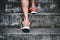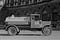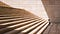# Stepping up: Image Classification using TensorFlow 2.x and TensorFlow DatasetPhoto by Bruno Nascimento on Unsplash

In this tutorial, we will build a network to predict the class of an image. Therefore we use the tf_flowers dataset, which consists of 3,670 and 5 different classes. This tutorial builds upon a tutorial by TensorFlow.

This tutorial follows our well-known workflow:

1. Loading the data and preparing our datasets
2. Define the model
3. Training and evaluating the model

# Loading the data and preparing our datasets

First, let's have a look at our dataset. We are using the tf_flowers dataset provided by the TensorFlow Dataset library (tfds).

We, therefore, load the dataset in our get_dataset() function using tfds.

# Predict fuel efficiency using an Artificial Neural Network in TensorFlow 2.xPhoto by Austrian National Library on Unsplash

After we started using just one linear neuron, we continue and build our first Artificial Neural Network (ANN). This tutorial is built upon the regression tutorial by Tensorflow.

In this tutorial, we are using a dataset describing cars. We are provided with data as the number of cylinders, horsepower, weight, etc. We need to predict the miles per gallon (MPG). Therefore we use the labeled training data and implement our first ANN.

In the last section of this tutorial are a few ideas that you could try to optimize further.

# Setting up the dataset

As last time we start by setting up the dataset…

# Gentle Start to Deep Learning implementing Linear Neurons using NumPyPhoto by Jukan Tateisi on Unsplash

When starting with Machine Learning and Deep Learning, the number of new vocabularies and concepts can be overwhelming. In this story, we tackle this problem by implementing a linear neuron using just NumPy.

A linear neuron highly relates to the problem of linear regression. The problem we try to solve is equal: We are applying gradient descent on our regression problem to find good weights w.

In this post, we look at the loss function, gradient descent, and training of our model.

# Steps to Train a Model

The steps to train a model can be divided into:

1. Loading the data and preparing our datasets
2. Define…## Jan Bollenbacher

I am a PhD Student in the field of Machine Learning, living in Germany